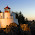## Friday, August 14, 2015

### How to find whether a triangle is Acute Angled Obtuse Angled or Right Angled with examples and Basics of Angular Bisector Theorem

In this video you are going to find the proof of why a triangle is an acute angled triangle or obtuse angled triangles or right angled triangle. The usage of formula given in books is proved here in this video in great detail and will let you know why this is used.

Also after explaining you the proof I have solved two questions in detail applying those particular formula and telling you about the short tricks to be used in the examination. The questions are -

Question 1. The sum of all the sides of a triangle with length in natural numbers  is 13. How many such triangles exist?

Also tell how many such

1. Isosceles triangles exist......
2. Scalene Triangles exist.....
3. Acute Angled Triangles exist.....
4. Obtuse Angled Triangles exist.....

Question 2. The sum of all the sides of a triangle with length in natural numbers  is 16. How many such triangles exist?

Also tell how many such

1. Isosceles triangles exist......
2. Scalene Triangles exist.....
3. Acute Angled Triangles exist.....
4. Obtuse Angled Triangles exist.....

At the end of the video a basic explanation about the Angular Bisector Theorem has been given. In the next video I would explain Angular Bisector Theorem and solve its questions and would take up apollonius theorem, its proof and questions based on it.

View more videos of Quant and Logic for CAT at http://gs-india.blogspot.in/2014/04/quantitative-aptitude-and-reasoning.html

1.2.